• Introduction
• Power Electronics
• SMPS
• DC Drives
• AC Drives
• Drives
• Green Energy

## Setting up a simple simulation using circuit components, block diagram components and library blocks.

In this tutorial, we will build three simple simulations by using circuit components, block diagrams and library blocks. As we know, all the circuit components in CASPOC are located in Components/Circuit and include plenty of components like resistor, inductor, capacitor, voltage and current sources and so on. The block diagrams are located in Components/Blocks and include plenty of signal sources and mathematical operators. The library blocks are located in Components/Library and include other complex models which is built by circuit components or block diagrams. When we choose different components from different categories, we shall also pay attention to the dots of each components in order to know if we can connect any two components or assign a label to certain dots. Round dots stand for the nodes which transmit voltage and current values during the simulation, and square dots for the input/output values of the blocks. In other words, the input/output unit depends on the properties of the blocks.

### Building a simple simulation of LED (light-emitting diode)

The first example is to build a simple LED circuit which includes one voltage source, one resistor and one LED as below.Because the performance of LED is temperature dependent, we will not only build the simple LED circuit but also compare the V-I curves under standard test condition (25 degrees) and higher temperature (125 degrees).

To build the above circuit, left-click VAC50HZ located in Components/Circuit/Sources and release the mouse button (step 1). Move the mouse cursor to the workscreen and left-click to put the VAC50HZ as below (step 2). Click 'OK' to include reference label 'Ground' or '0'.Then we will see there is a label 'ground' in the cathode of VAC50HZ. To select a resistor, left-click R located in Components/Circuit/RLC and release the mouse button (step 1). Move the mouse cursor to the workscreen and left-click to put the resistor as below (step 2).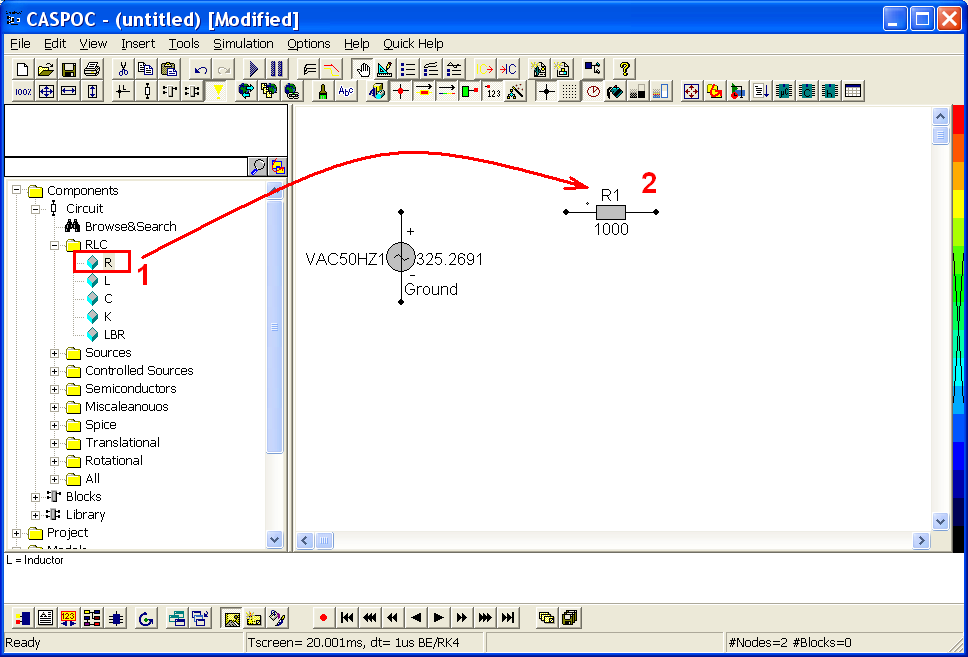To select the LED, left-click the LED component in Components/Library/Lighting/LED and release the mouse button. Move the mouse cursor to the workscreen and left-click to put the LED as below.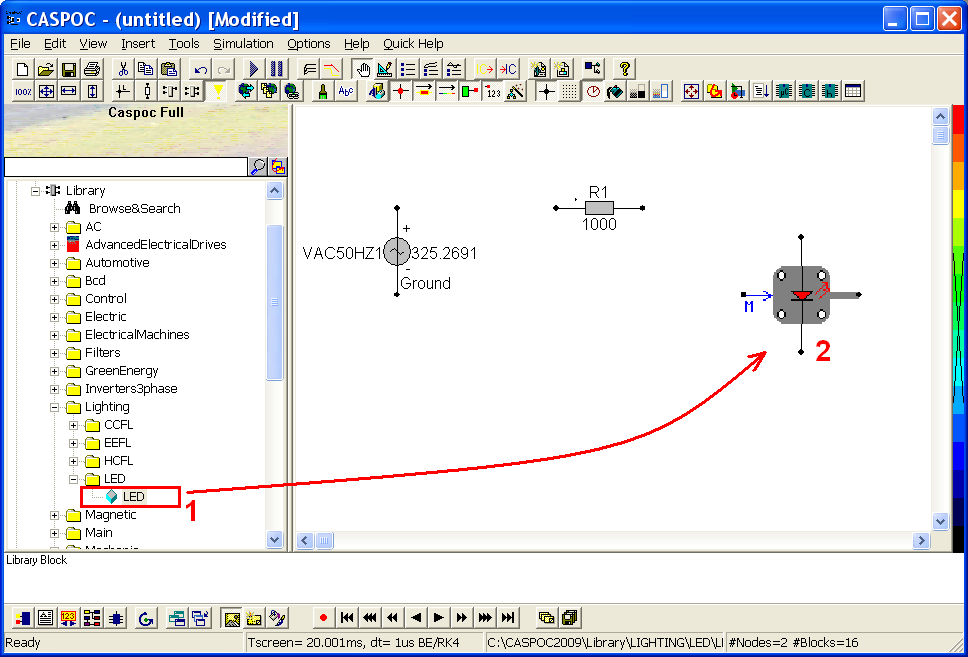Connect the three components as below (see the red line). Note that there is only one 'ground' label and in the cathode of the voltage source VAC50HZ. If not, please correct it by right-clicking the node and change the label value. In CASPOC, the label '0' is equal to the label 'ground' which stands for the reference point in an electrical circuit. You can also replace the label 'ground' to '0' for lazy type. If you need some specified symbols for ground, you can go to Components/Library/Electric/Ground for selecting the one you like.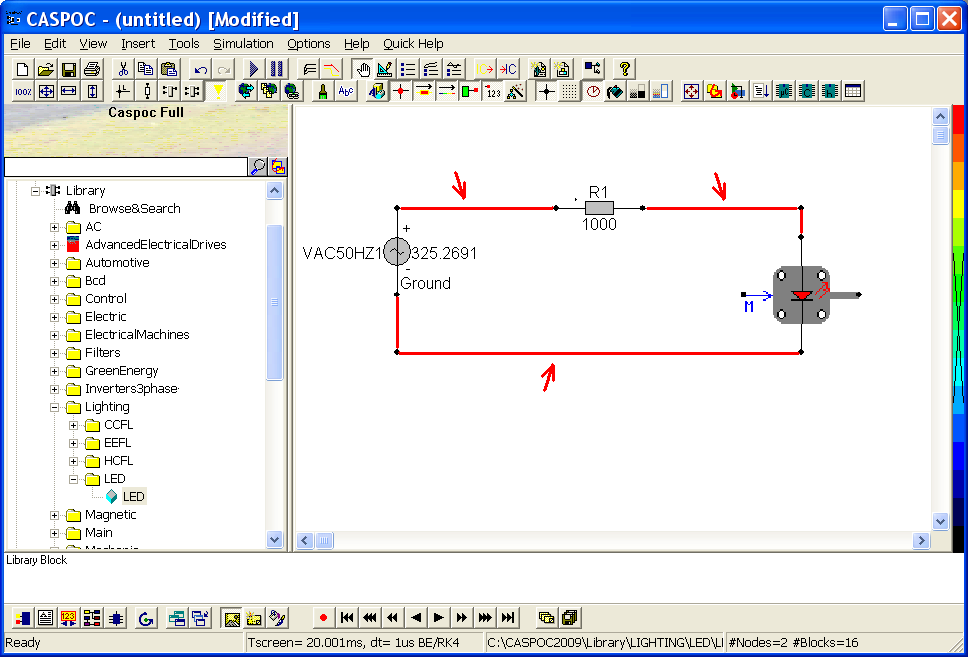After we have all the basic components, now we can start to configure their parameters. Right-click the voltage source VAC50HZ (step 1) and set the value to 20 volts (step 2). Right-click the resistor R1 (step 3) and set the value to 1 ohm (step 4). Right-click the LED component (step 5) and verify if the parameters are the same as below: Cth=0.5m, Initial Temp=25, Rth=1, alpha=0.7, dVdT=-2m (step 6).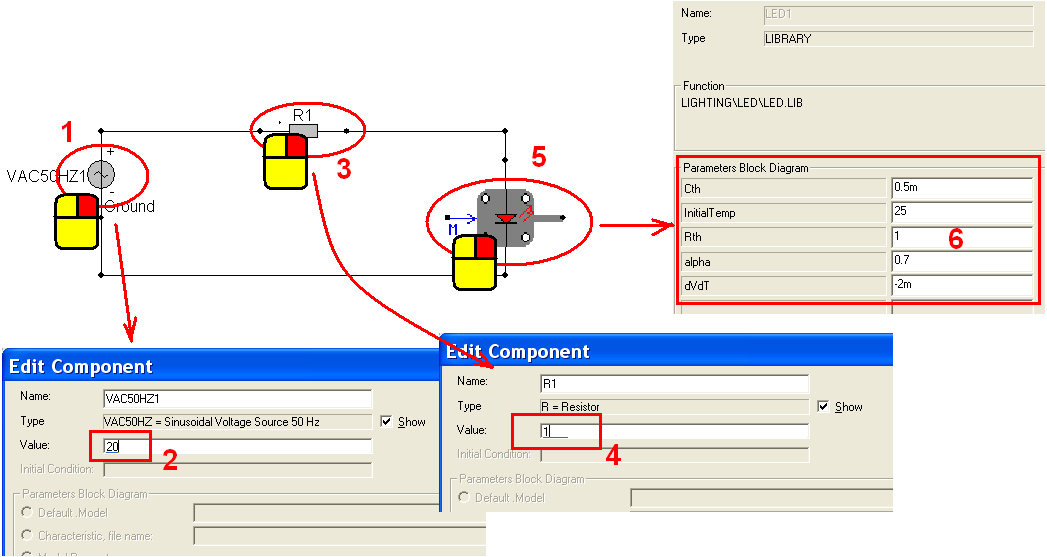Then we can assign the property of the LED component by using 'filename'. Left-click the FILENAME located in Components/Blocks/Miscellaneous and release the mouse button (step 1). Move the mouse cursor to the workscreen and left-click to put the FILENAME in the left of the LED (step 2).Connect the FILENAME and LED (step 1). Right-click the FILENAME and find a popup window (step 2).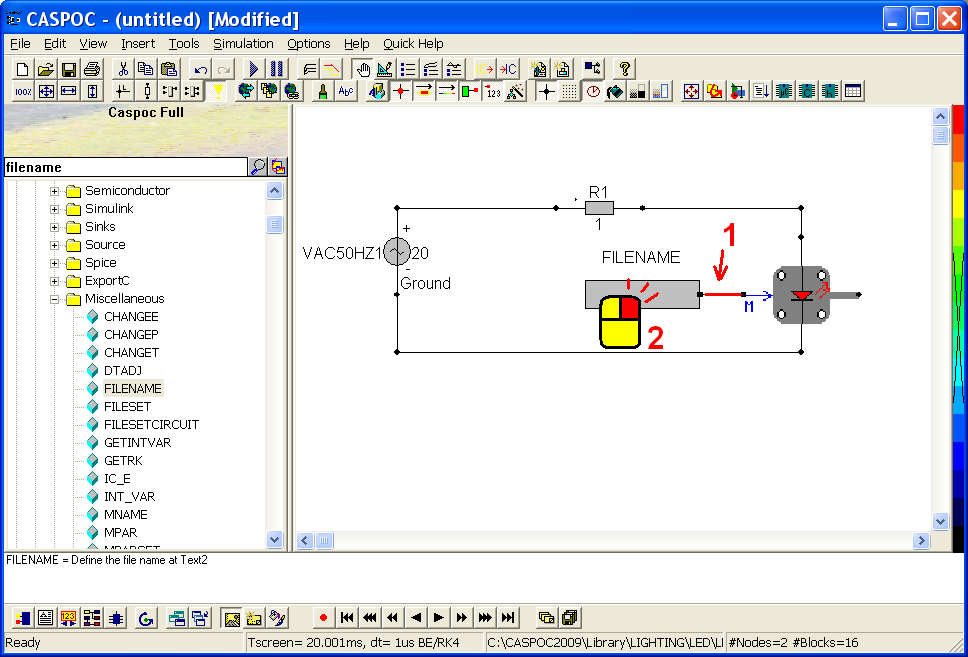Define the V-I curve in Text1 (step 1) which you can also enter by clicking 'Edit' button in the right side of Text1. Click OK after finishing. Note that the V-I curve is [0.4 0.01, 0.5 0.25, 0.6 1, 0.8 4, 0.9 9] under stand test condition 25 degrees. In other words, it must stand for the V-I curve when your LED works under 25 degrees.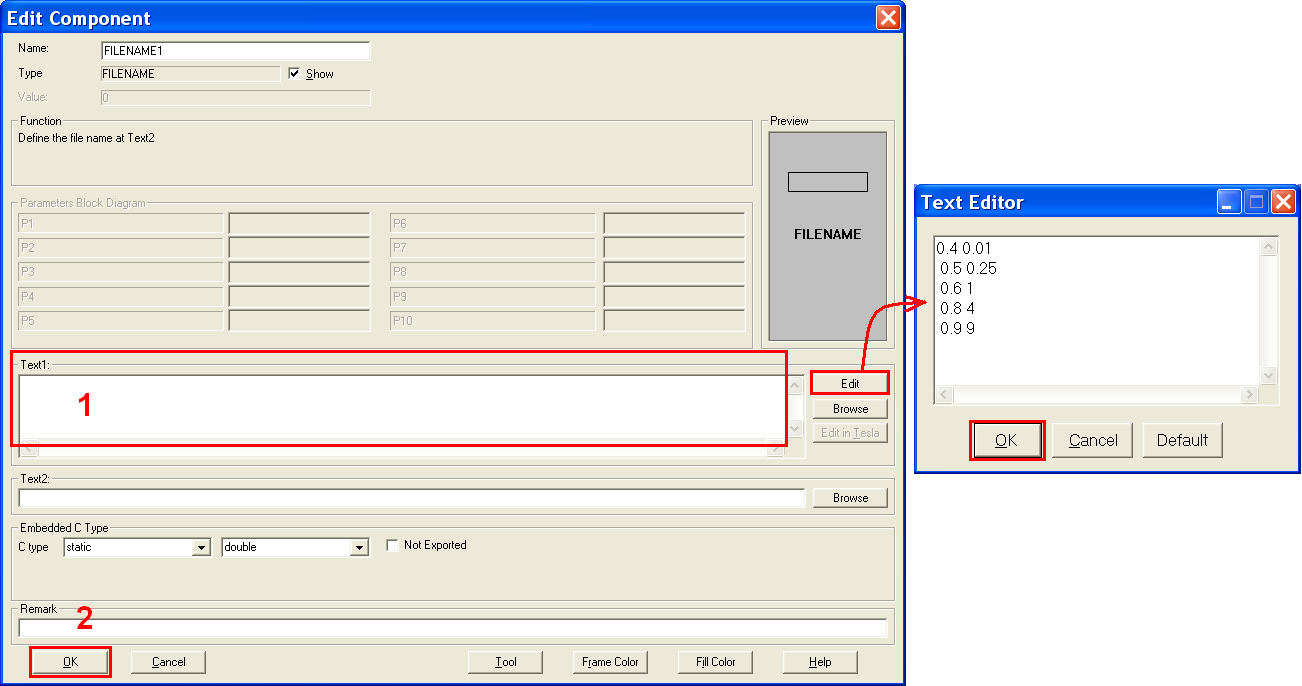Since we have all the necessary components, we can start to define the environmental temperature of the circuit. Left-click the ambient component located in Components/Library/Thermal/Sink and release the mouse button (step 1). Move the mouse cursor to the workscreen and left-click to put the Ambient in the right side of LED and connect to it. Right-click the Ambient component (step 2) and set the temperature to 25 degree (step 3).Use a SCOPE to observe the voltage and current values of the circuit. Click the button to insert a scope in the right side of the LED circuit and enlarge it by dragging the right-bottom of the SCOPE as below.Connect the anode of LED to the first yellow input trace of the SCOPE (step 1) to measure the voltage value of LED. Right-click the second red input trace (step 2) and type Trace=Current through: R1 in the popup window (step 3) so that we can measure the current value of the LED.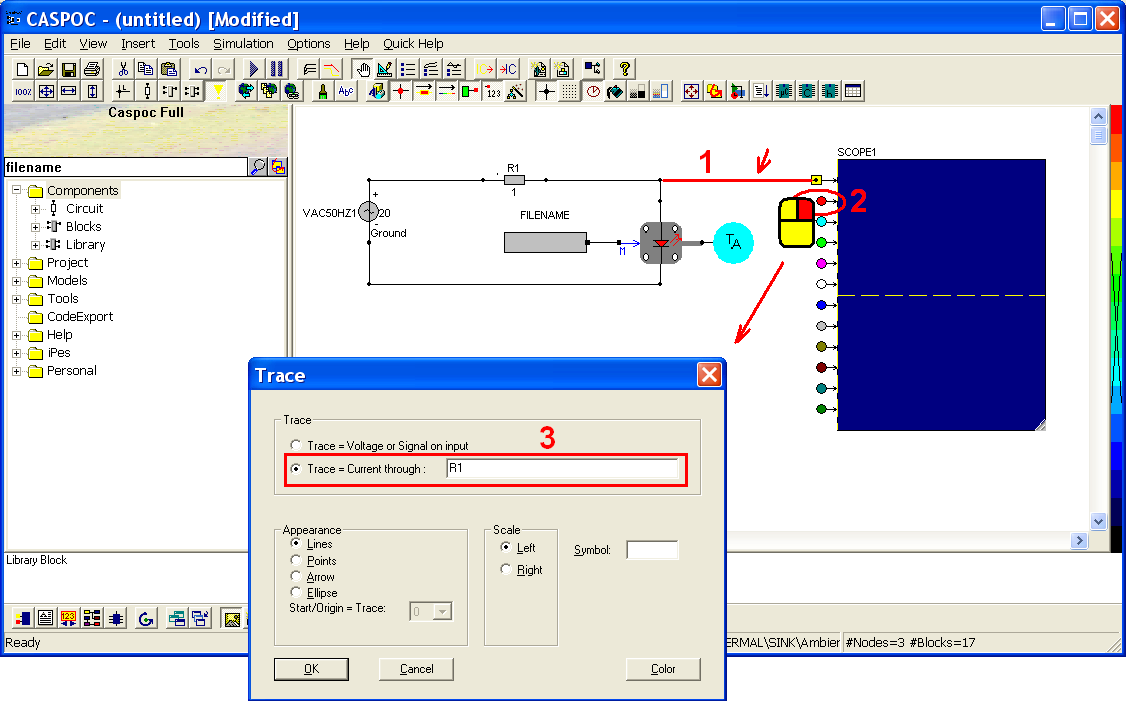Configure the simulation parameters by clicking the shortcut (step 1) or go to Simulation/Simulation Parameters in the menu bar. Set up the parameters to Tscreen=10.1m and dt=10u (step 2). Make sure the simulation parameters as below and the numerical integration method circuit = Euler. Click OK if there is no mistake.Basically, the default setting of the SCOPE is to acquire and display the simulation results according to the time varying. Now in order to display the V-I character curve, right-click the SCOPE (step 1) and select View/XY Writer (step 2) to set voltage value as X-axis and current value as Y-axis. In CASPOC, when you select XY writer, the odd input traces will be mapped to X-axis, and the even input traces to Y-axis.Now we can try to execute the LED circuit by clicking the shortcut (step 1) or select the Simulation/Start Simulation in the menu bar. Then observe the simulation result in the SCOPE (step 2). We can compare the simulation result to the FILENAME which we just set up, then we will find the simulation result is absolutely the same as what we defined under 25 degrees.### Compare the performances of LED circuit under different temperatures

But our goal in this tutorial is to compare the different V-I curves under different temperatures. Now we can start to add the second circuit which is the same as previous one but under a higher temperature. Left-click a resistor in Components/Circuit/RLC (step 1) and move the mouse cursor to put the resistor R2 under the first circuit. Right-click the resistor R2 (step 2) and change the value to 1 ohm (step 3).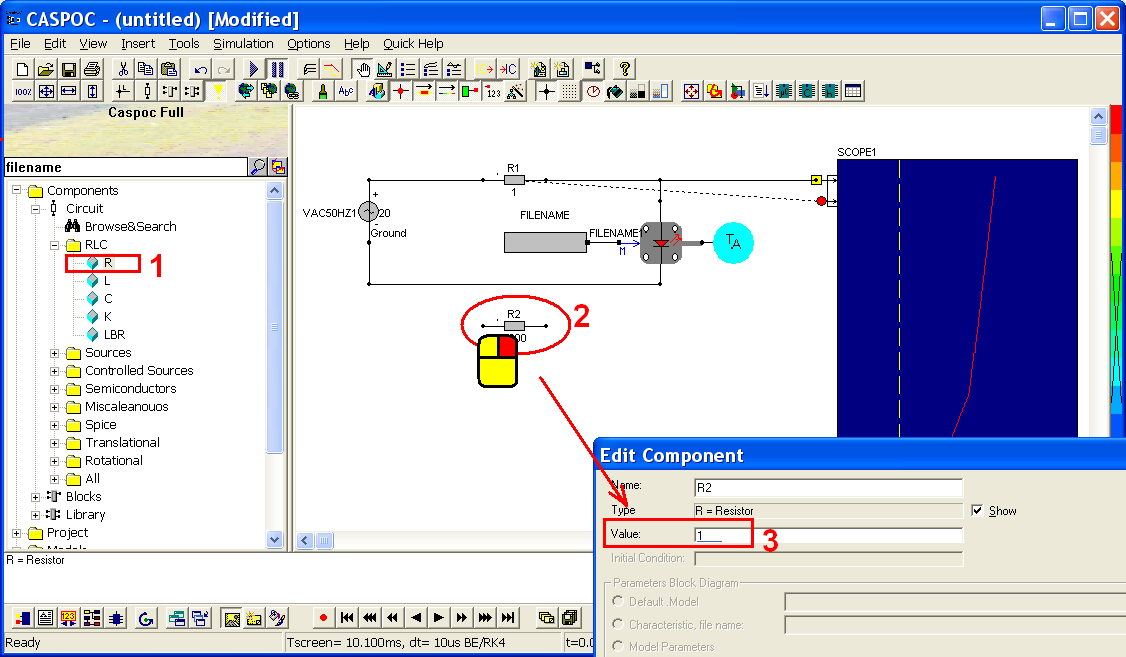Similarly, left-click the LED component in Components/library/Lighting/LED (step 1) and release the mouse button. Move the cursor to the workscreen to put the LED under the first LED as below (step 2).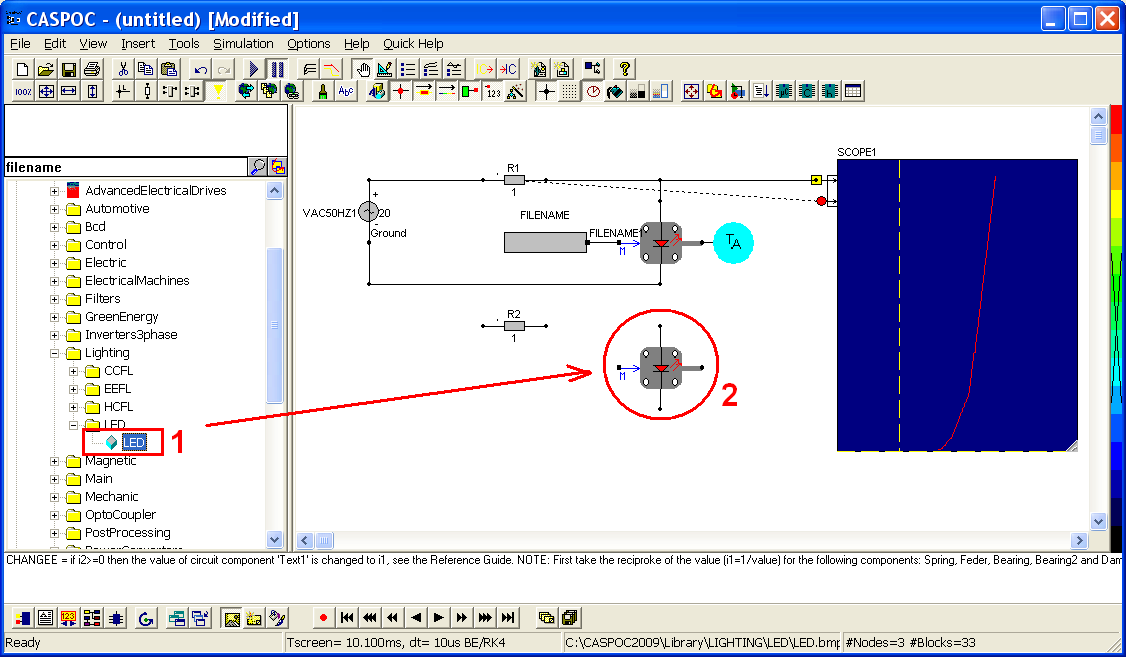Left-click the ambient component in Components/Library/Thermal/Sink (step 1) and release the mouse button. Move the cursor to the workscreen to put the ambient component in the right side of the second LED component and connect to it. Right-click the LED component (step 2) and change the value of the temperature to 125 degrees (step 3).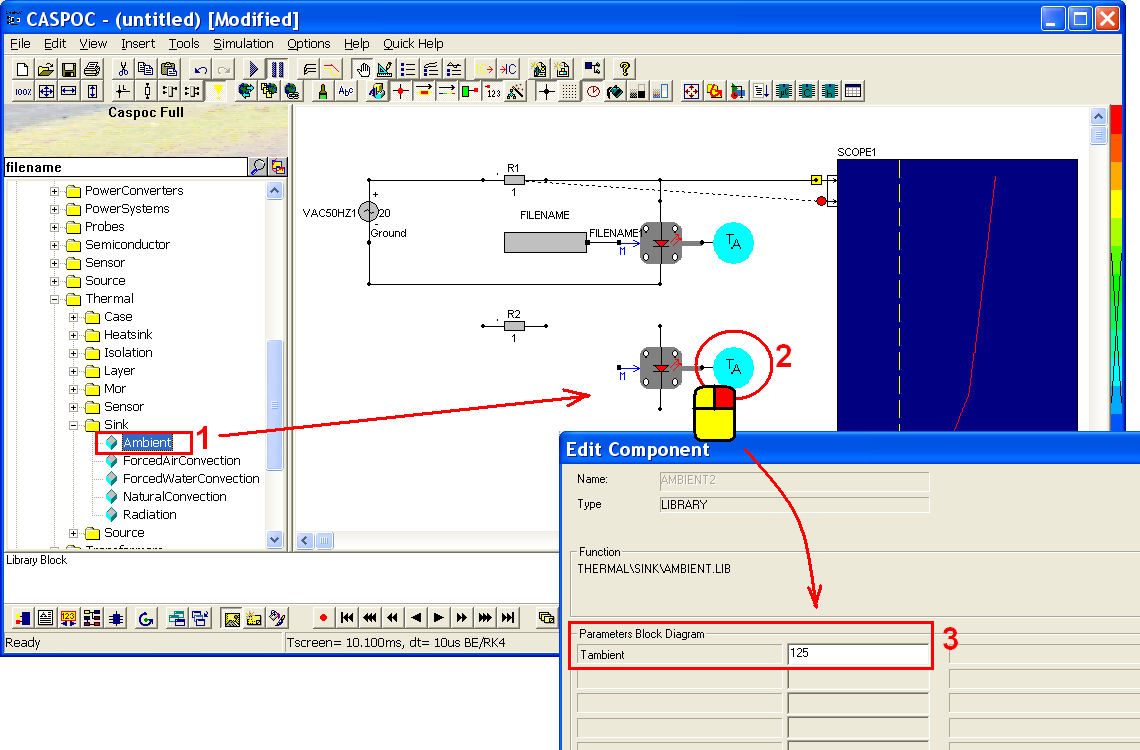To share the same voltage source, connect the resistor, LED and ambient components as below (red line): The anode of VAC50HZ connects to the anode of the resistor R2; the cathode of the resistor R2 connects to the anode of the second LED; the cathode of the second LED connects to the cathode of VAC50HZ with label 'ground'.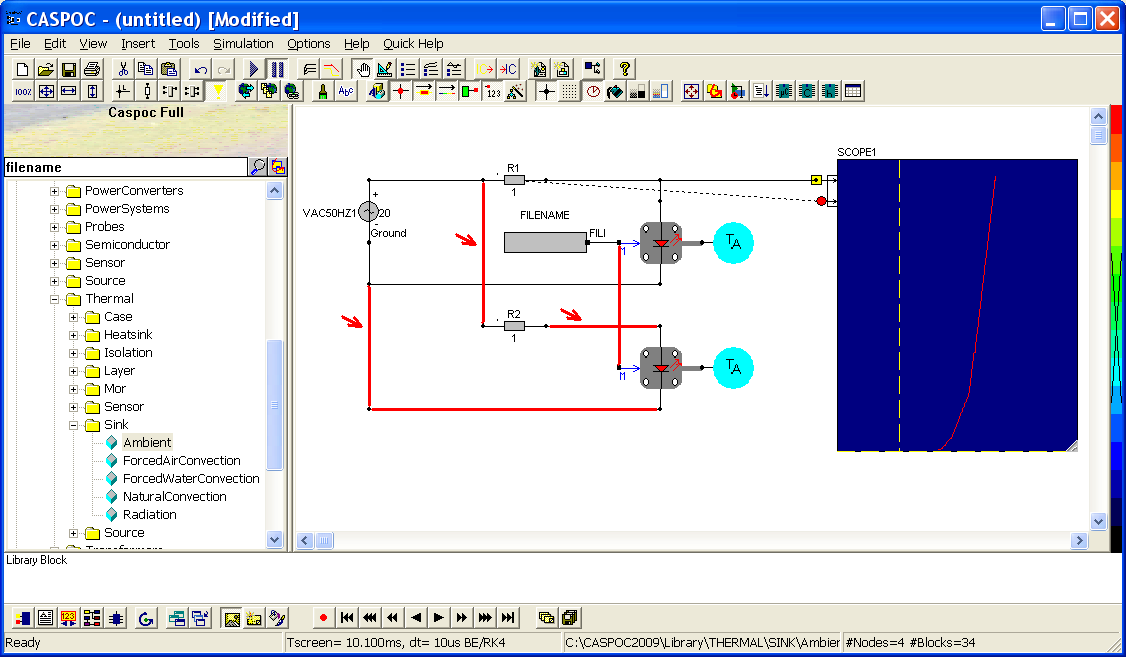Connect the anode of the second LED component to the third blue input trace (step 1) to measure the voltage value of the second LED. Right-click the fourth green input trace (step 2), and set Trace=current through: R2 (step 3) to measure the current value of the second LED.Then execute the LED circuit by clicking the shortcut (step 1) or select the Simulation/Start Simulation in the menu bar. Then observe the simulation result in the SCOPE (step 2). We can see the red curve is the first LED circuit under 25 degrees, and the green curve stands for the second LED under 125 degrees.You can also find this example in the folder of CASPOC: \\CASPOC2009\Samples\Lighting\LED\LED_StaticTemperatureDepending.csi

### Using multisim for batch experience

In the previous exercise, the workscreen which we worked on is the main schematic. Go to Project/Schematic/Main Schematic and then find there are also all the library blocks under the same tree view. Click 'Ambient1' and we will see the schematic of the library in the right side. In this library Ambient, it's built by one controlled voltage source B1. The anode of B1 shows the output voltage in the left side of the Ambient and affects another LED component. We already did this part in this tutorial. The cathode of B1 with label 0 is the reference point 'ground'. The controlled signal for B1 is a block diagram 'MPAR' which stands for model parameters and can read the value which users set in the parameter pop-up window. From above, we can know the Ambient is like a controlled voltage source for LED circuit and we will replace the ambient by using a controlled voltage source in the following steps.Go back to the Main Schematics from the Ambient1 by clicking (step 1). Delete the second Ambient from the original circuit as below (step 2).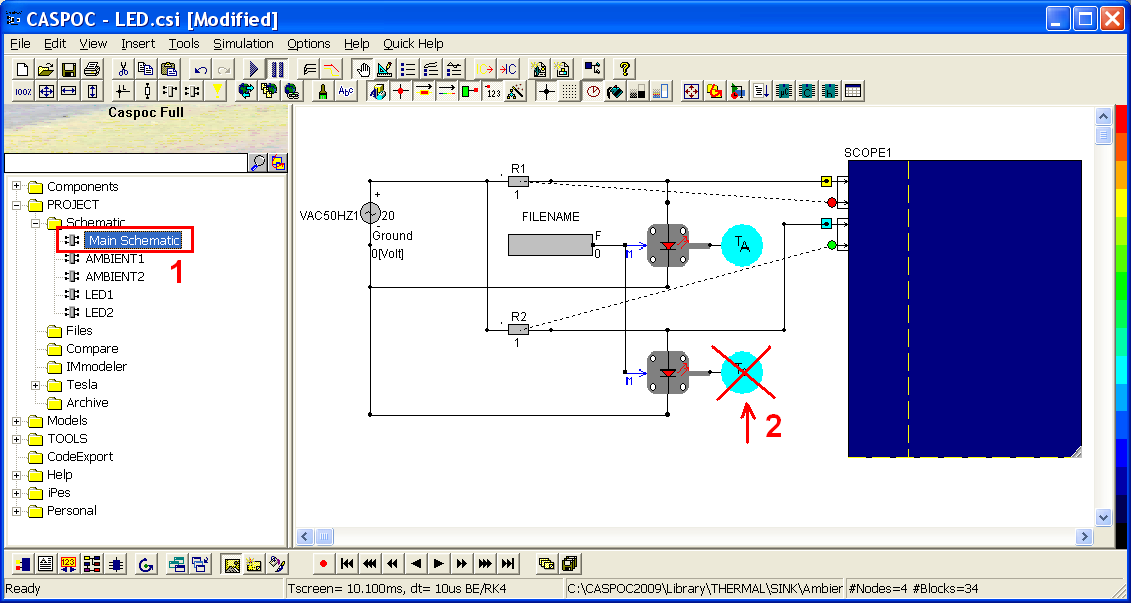Left-click the controlled voltage source B from Components/Circuit/ControlledSources (step 1) and release the mouse button. Move the cursor to the workscreen and put the B1 in the right side (thermal input) of the second LED and connect to it (step 2). Note that the thermal input of LED is round dot, it shall connect to the anode of B1 which is round dot too. Keep the square dot (controlled input) downward and we'll define its input.Right-click the square dot of B1 and enter the label value to 125 which stands for the environment temperature 125 degrees.Right-click the cathode of B1 and enter the label value to 0 which stands for electrical ground.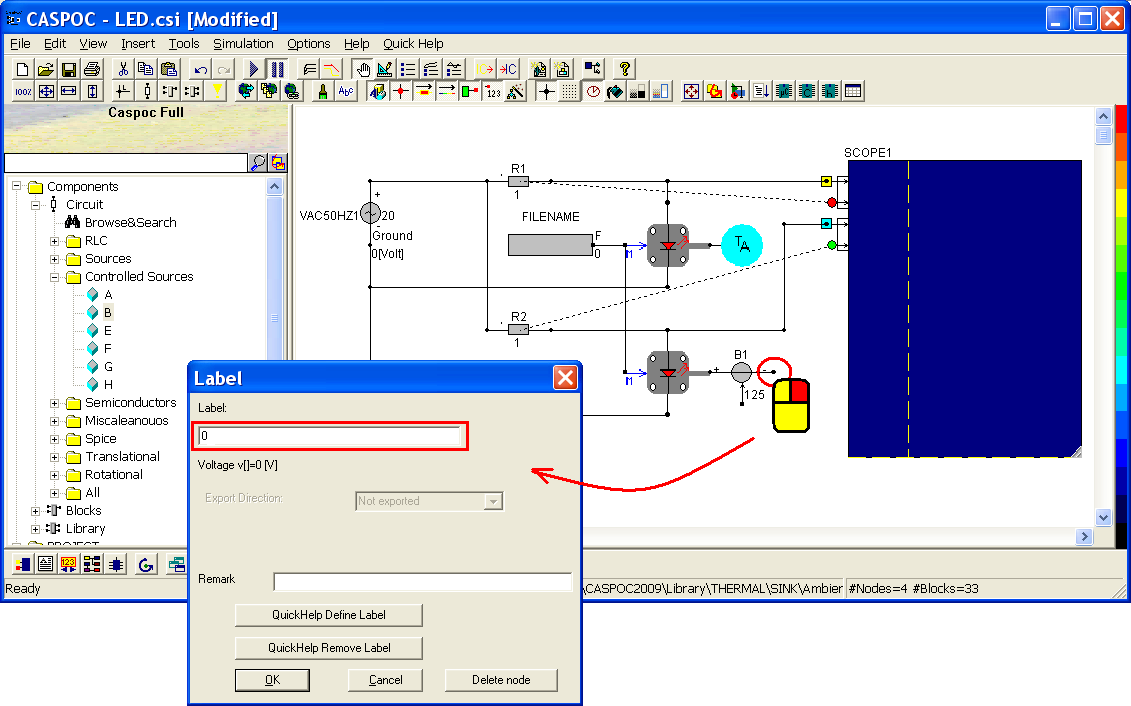Then click the short button to execute this simulation (step 1) or go to Simulation/Start Simulation in the menu bar. Observe the simulation result and compare it to the previous one with two Ambient components (step 2).Then delete the components which is used for the second LED circuit, like the resistor R2, LED2 and the wires which are not necessary (step 1). Also delete the Ambient1 and only keep the controlled voltage source B1. Remove the label value of B1 by right-clicking the square dot (step 2) and set no value in the label (step 3).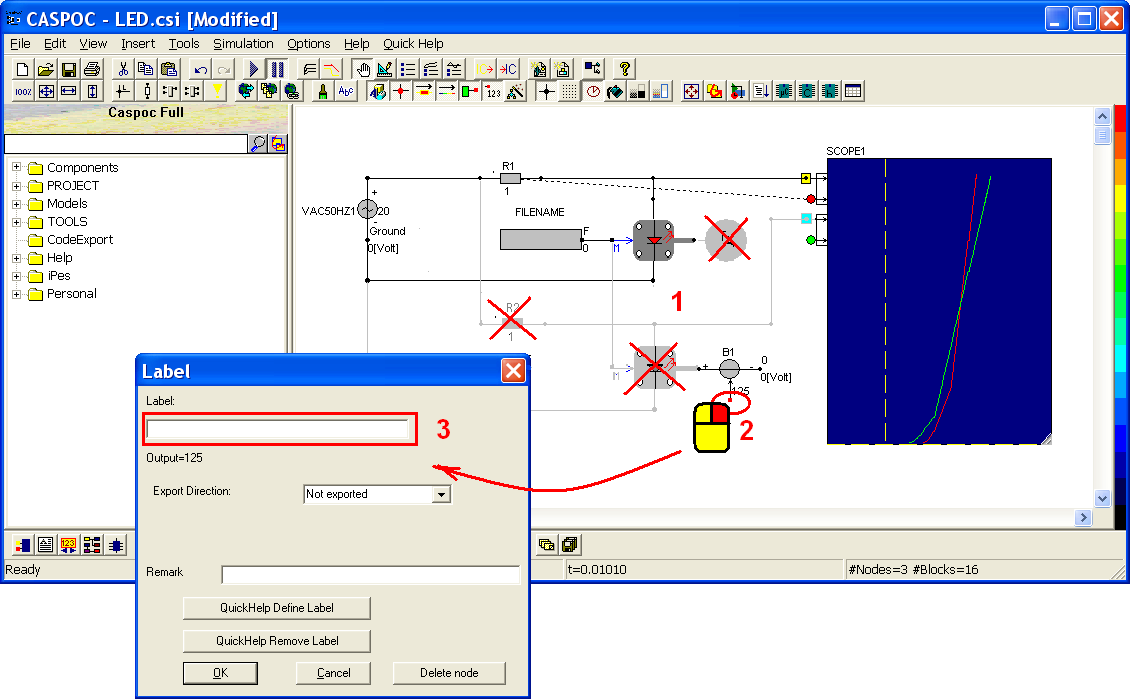Move B1 to the right side of LED and connect the anode of B1 to the thermal input of LED (step 1). Left-click Multisim and CON in Components/Blocks/Source (step 2) and release the mouse button. Move the cursor to the workscreen and put Multisim and CON as below. Here Multisim stands for multiple simulation and CON stands for constant.Left-click ADD and GAI in Components/Blocks/Math and release the mouse button. Move the cursor to the workscreen to put the ADD and GAI as below. ADD is a mathematical operator for addition. GAI means gain which will multiple all the input value and then send to the next block diagram.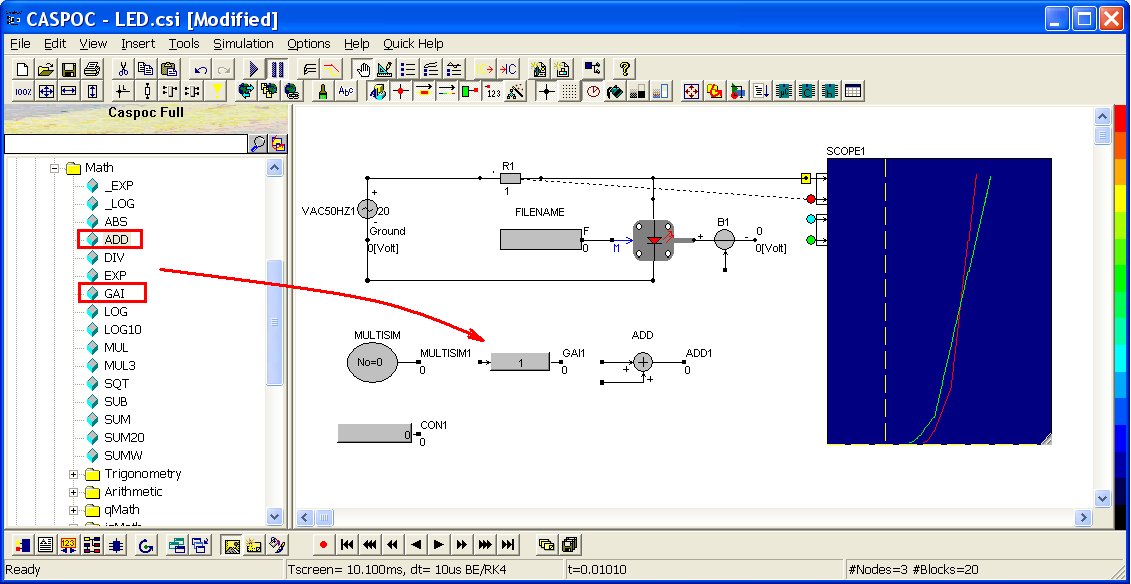Connect the four block diagrams as below and give the calculation result to be the controlled voltage B1. Multisim depends the number of simulation to send the value 0, 1, 2, 3,....until the maximum. For example, if you set the simulation number to 4, Multisim will generate the value 0, 1, 2, 3, 4 for simulating five times. So, we can know here we make a operation: Controlled voltage = CON+ GAI*No, where No stands for the value of Multisim.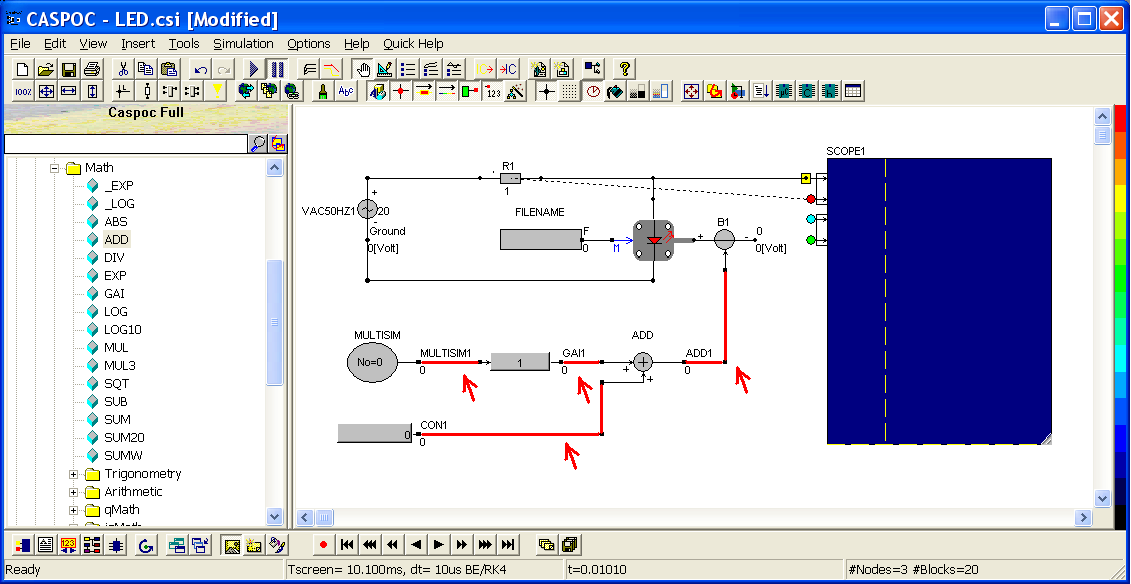Now give the value of CON and GAI by right-clicking the components. Enter CON=25 and GAI=25 so that Controlled voltage = CON+ GAI*No= 25 + 25*No, where No = the value from Multisim. We just need to control the times of simulation, then we can control the controlled voltage and ambient temperature.Click the shortcut of multiple simulation parameters (step 1) or go to Simulation/Multiple Simulation Parameters in the menu bar. Set the number to 4 and click OK.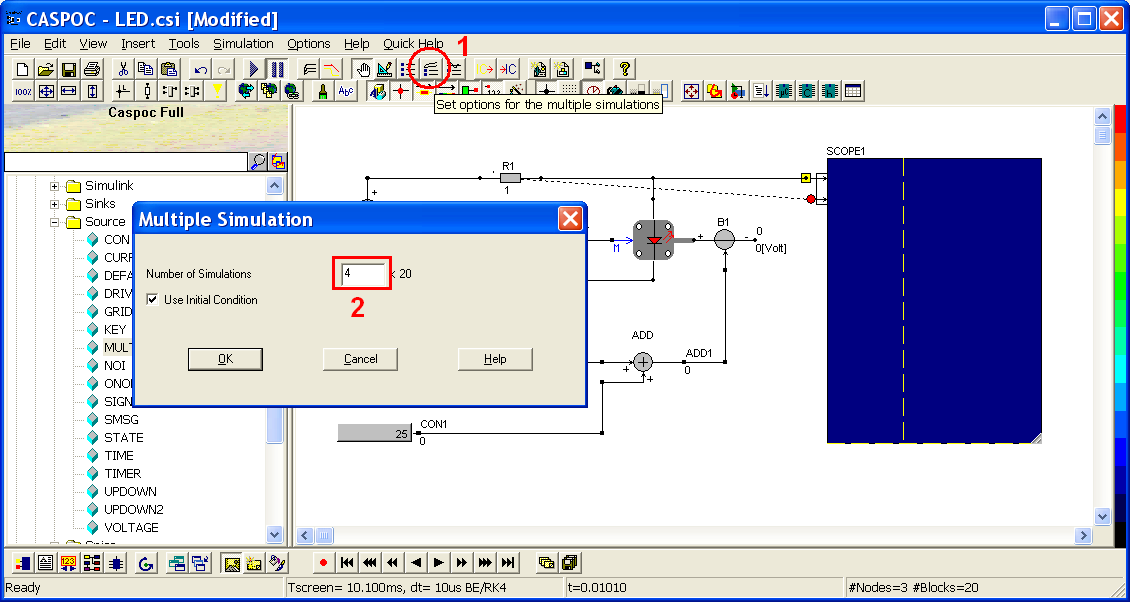Then execute the multiple simulation by clicking the shortcut of multiple simulation (step 1) or go to Simulation/Start Multiple Simulation in the menu bar. Observe the simulation result in SCOPE and compare how the V-I curve changes which the ambient temperature changes (step 2).